# How to use Analog Multimeter

Hi blogger fans, In this blog post we will learn about analog multimeter we will study their principle of working and we will see how to use analog multimeter in mobile repairing for measurement of voltage, current, Ohms, Resistance, and Continuity or Beep Testing. so let us start learning.

# What is Analog Multimeter

Engineers around the world trust analog multimeter for high-quality measuring. because the major part is designed to withstand shock. The fuse protects the meter. Even when a voltage up to AC 230 volt is imposed on each range for type 5 seconds.

## How to Read a Analog MultimeterHow to read analog multimeter

If you are a beginner, then how to read an analogue multimeter basic is written in detail with pictures in this article about analogue multimeter how to use. But if you know how to read analog multimeter scale, then the maximum DC voltage that you can measure with this multimeter analog is 1000 volts. You can measure 10 microfarads of Capacitance with this meter. Use the optional probe analog multimeter function for measuring DC high voltage like 25 KB. you can measure a maximum of 1000 AC high voltage if you know how to measure AC voltage using analog multimeter. Before we start learning how to use a multimeter analog, the first thing we should know is the analog multimeter scale reading. In the mobile repair course, the teacher first teaches students to read voltage in analog multimeter, then later in the course to trace faults on the mobile PCB. He teaches to read multimeter readings.

This video on analog multimeter is how to use to help you learn how to use analog multimeter in mobile repairing order a hard copy of how to Use Analog Multimeter in Hindi book for the mobile repairing course so watch it until the end. If you know the uses of analog multimeters, it is important to know what type of multimeter you need before you buy one as they come in many varieties like analog multimeter and analog multimeter tester. For example, some of them are designed for use with low-voltage circuits, while others are designed for high-voltage circuits. analogue multimeter diagram gives you exposure to what your analog multimeter ohms do. To fulfill this need, buy the book on analog multimeter use. read multimeter analog sanwa

Analog multimeter is most commonly used by mobile engineers and technicians in the mobile phone workshop and for the repair of mobile phones and smartphones.Best analogue multimeter

Now this best analog multimeter, as the name suggests, is a multimeter multi means many and meter means measurements, so this analog multimeter is an instrument that is used for making
Multiple measurements on the mobile phone and its PCB. various types of measurements can be done with analog multimeter sanwa. these measurements include the measurement of DC current and resistance in mobile repairing. the analog multimeter can measure online voltage and offline resistance of various ranges on the mobile phone PCB. That is the best analog multimeter definition. Now let us understand how we can use the analog meter and what are safety features that you need to keep in mind.

This Galco TV video cover analog multimeter basics and show you some advantages using analog multimeter have over digital multimeters

if you have an analog multimeter already that's good or if not! use this link to find analog multimeters that suit your price range

## Analogue and Digital Multimeter Difference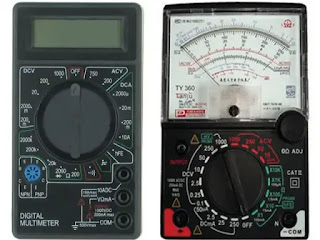Advantages and disadvantages of analog and digital multimeter

As we know there are two types of multimeter.
1. digital multimeter
2. analog multimeter
The difference between these two multimeters is that the digital multimeter is going to give us the output in the digital form whereas the analog multimeter gives the output in the analog form. Today we live in a digital age and mobile phone technicians always wonder why do I need to use an analog multimeter to diagnose faults in mobile phone PCB when they can have a digital multimeter well there are some things that an analog multimeter can do that an digital multimeter can't. I hope you understand the basics of What is multimeter and its types? and we'll demonstrate that as we go through this article so read it completely. To give readers full benefits download analog and digital multimeter pdf. the article is along with how to use multimeter pdf.How do you use a digital multimeter?

What is the difference between analog and digital multimeter? To know the answer we have a complete step-by-step guide on a digital multimeter. Click here to read How do you use a digital multimeter

Whereas analog output means that we will be having a pointer will be moving over a scale on the scale we will be having divisions and with the help of reading that scale, we can get the output of the instrument so here the output is analog.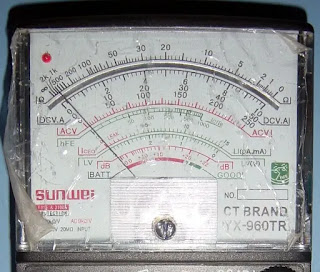Sanwa multimeter

Now we know that for the measurement of voltage, the instrument which measures voltage is called the voltmeter. below in this article, we teach you to read a voltmeter.Voltmeter amazon

the instrument that measures current is called an ammeter.Definition of ammeter

and the instrument that measures resistance is called the ohmmeter.Ohmmeter definition and function

Because the volt is the unit for voltage, the unit for current is ampere and the unit for resistance is ohms so based on that instruments are called voltmeter ammeter, and ohmmeter.

If you want to know cell phone multimeter read how to use mobile repairing tools to repair mobile phones this page gives you a cell phone repairing tools Review and a pdf guide on cellphone repair tools

### Analog Multimeter Probes

You need to understand what probes are. probes are also called analog multimeter leads. As you can see in the picture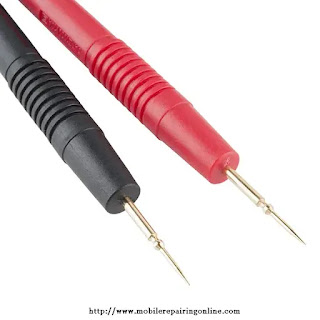Fluke multimeter probes

Two probes are commonly used with all analog multimeters. There will be two leads one lead is red in color and the other lead is black in color. so red is taken as for the positive connection and black is taken as negative or analog multimeter use we will be having two leads red and black.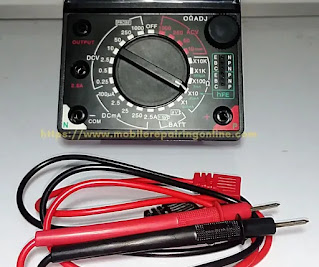Silicone test leads

We have to plug in our test leads your red test lead goes into the positive Jack and your black test lead goes into your negative Jack. I hope you get the answer to Where do the leads go on a multimeter? when you see this pictureFluke test leads

We're going to discuss how to read this scale we're going to talk about how to read analog multimeter, What is the function of analog multimeter, How to measure voltage with an analog multimeter, how to read an ohms value, and how to read DC volts and AC volts. Before we start reading analog multimeters and know What is analog measurement? and How do you measure on analog meter? let me show you the first measurement scale on the analog meter.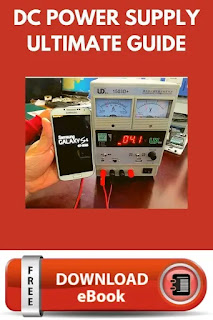DC power supply mobile repairing

Read Mobile DC Power Supply A Complete Step-by-Step Guide to Know the Testing Principle and uses of dc power supply

#### Analog Scale MeasurementsAnalog multimeter function

This is what we call the measurement scale on the analog meter. Now let us understand what these other readings are displayed on the measurement scale of analog multimeter. Now, on the left-hand side, you'll see something written as DCV.  This is the symbol of DC Current in the analog meter. when you turn the meter knob to the left you will able to use the dc voltage meter on this Sanwa multimeter.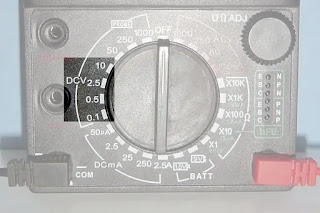How to read DC voltage on an analog multimeter

Where the range is between 2.5Volts to 1000 Volts. you can check the DC voltage by placing the knob at the respective voltage point. If you have a smaller voltage to be checked you can place it at 10Volts and higher Voltages you can go on up to 1000 Volts to measure DC Voltage. On the right side of the meter, you see written ACV. This is the symbol of AC Current in the analog meter. When you turn the meter knob to the right you will able to use the ac voltage meter in this fluke analog multimeter.How do you check AC voltage with a multimeter?

Where the range is between 10Volts to 1000 Volts. you can check the AC voltage by placing the knob at the respective voltage point. for AC smaller equipment that requires 200 Volts or less, you can place a meter knob at the 250 volts point, and for Higher value, AC equipment you can measure using the 1000 Volts point. you can select ACV you can select DCV. AC means alternating current and DC means direct current. you can't get AC value on DCV. DC value on ACV.

The fuse protection on your meter gets infected. so be careful when you set your meter measurement scale. I hope you understand what is ac and dc voltage testing with an analog multimeter. these are the two most important ways of measuring AC and DC voltage using an analog multimeter. some mobile technician calls measurement scale a knob or dial.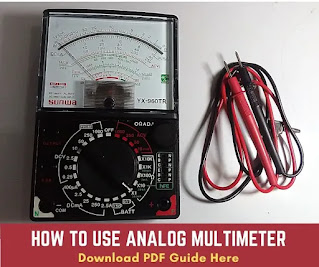Using analog multimeter

Analog multimeter Amazon pdf guide gives you understanding analog multimeter principle in the Area of mobile repairing analog multimeter probes, how to read analog multimeter, and learning How to measure AC voltage, DC voltage, Ohms, Resistance, and Continuity Testing in the mobile phone repairing field. what are you waiting for download how to use analog multimeter pdf today?

## How to read DC Voltage on an Analog Multimeter

You can read the battery voltage on the label of the mobile battery. Most mobile phone batteries are 3.7V or 3.8Volts. For how to use analog multimeter to test the battery place the analog multimeter knob on DCV 10 volts. leads will be connected with the battery terminals positive to battery positive and negative to battery negative. follow the picture below to touch the probes of the multimeter on the terminal of the battery.How is DC voltage measured?

The meter shows a reading on the scale. is slightly from the AC scale. follow the picture below for analog multimeter reading.

If the reading scale on the meter shows more than 3.7 volts then the battery is fully charged. And if it is less than 3.7 volts you will have to charge the battery with a charger. so that's how you read the DC scale here.DC voltage reading

Note: If the battery is drained and showing 0 voltage the battery is dead.

Read the article on how to test a cell phone battery with a multimeter to understand how a Battery Booster works where the Battery Booster probes need to be placed on the Mobile Phone Battery to repair it

if you want to know the following topics on how to use analog multimeter to measure current:
• how to use analog multimeter to measure voltage
• how to use analog multitester
• how to read an analog voltmeter
• analog multimeter working principle
• analog multimeter block diagram
• analog multimeter tester
then this analog voltmeter pdf is for you. the presentation help you know more about Measuring DC Voltage.

### How do you check AC Voltage with a multimeter?

When you want to test and read a voltage if you don't know the voltage always select the highest scale but if you know your voltage select the appropriate scale, for alternating current examples, let's say I was going to work on 220 volts I would select the 250-volt scale put it on my AC if that's what we'reAlternating current examples

going to read first and that is this scale right here the red one. so that's how you read the AC scale here.
when AC voltage measurement is to be done then again we have the two leads. place the meter knob on 250 volts ACV. One lead is connected to the AC phase and the other lead is connected with a neutral wire on the electric socket. meter show reading on the reading scale. we will connect this to the main power plug to check the voltage. in your room, some switches are available on the board. for checking AC Volts you can plug points on this board.How to measure AC voltage

Now, going further down on the right-hand side of the analog meter you'll see a few more micro readings that are multimeter resistance measurement principles. However, let us focus on only those readings that are very important during mobile repairing that we commonly take using an analog multimeter. Now let me show you how to measure ohms resistance on a multimeter to get a reading in the felid of mobile repairing.

#### How to read ohms on an analog multimeter?

Right below the AC voltage, you'll see a few Ohm readings given there. This is where you place the dial to check the value of resistance.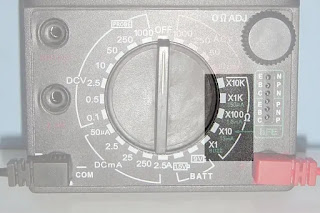How to measure resistance using an analog multimeter

When you check basic electronic components which range from 10 Ohms to 10 Kilo Ohms and that is how you turn the knob there you can place it at the respective point and check the resistance value.

How can mobile components use a digital multimeter? and How do you know if PCB components are bad? the answer is in How to Check Polar Capacitor blog postWhen the pointer of an analog ohmmeter

this is an analog meter reading scale. about reading the Ohm value. if you look at the scale here the very top scale is yours owns zero ohms here which means there's no resistance.How does a multimeter measure resistance

and when you come over to here this is infinity ohms.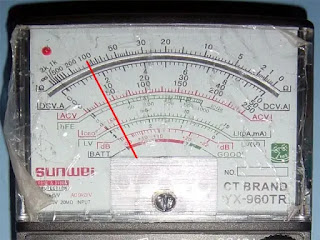Analog ohm meter

then we'll take our ohmmeter range switch and bring it over to the highest range our times 10,000 are being resistant and then we'd put our test probe together now the needle is going to fluctuate hereHow to test continuity with analog multimeter

what we want to do is we want to zero this out so we have our zero ohm adjuster dial here. also called zero ohm adjustment knob.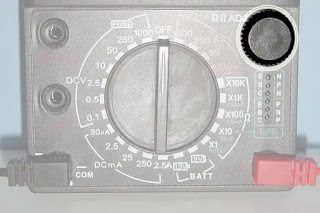Zero ohm adjustment knob

you want to bring the zero ohm adjustment knob down until it zeros right to there and that way you've zeroed out your meter. the answer of How do you zero an analog ohmmeter.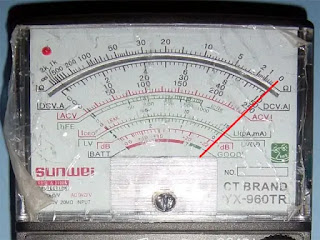Multimeter continuity symbol

Now you have a question. What is the purpose of the 0 ohm adjust knob on an analog meter?Analog meter

well if I want to get an accurate resistance reading I have to calibrate it the reason I have to calibrate the meter is that there's a battery in there and as the battery weakens it's going to change the values that it reads so now once we've got our meter zeroed out we can pick a range scale to go on depending on what we think that ohms value is. What does a high ohm reading mean? What is the purpose of the range selector switch?Range selector knob

we have our x one so if I came up to here and I was on the 10 that would be 10 ohms when I'm on our x one if I was our x 100 that would be a thousand ohms and if I was our x 10000 that would be 100 thousand ohms so this is how you read it from right to left on the top. reading ohms value on the analog meter. the answer What is the purpose of the range selector knob on analog multimeter.
I hope now you have your two questions answered in this article how does a multimeter measure resistance and How to read resistance on a multimeter. To read resistance in analog multimeter first turn on your meter to the resistance setting. most analog multimeters have multiple ranges.

## How to Measure Resistance Using Analog MultimeterStandard resistor

For example, to Measure Resistance using Analog Multimeter, we have a standard resistor.  let's say we don't know or we cannot see the color bars on the resistor so we can use o meter to measure the resistance of this unknown resistor. my advice to my reader is to make this easy and make sure you get stable connections. use hook alligator leads. use this link to buy alligator leads.Alligator leads

It helps you see stable reading on this type of resistance. To know Analog Multimeter Basics and Measuring Resistance (with Pictures) we have the two best PDF guides and PowerPoint Presentation free download for you. This pdf also shows the analog multimeter diagram. yes, a block diagram of an analog multimeter that helps you know more about old analog multimeters. The PDF direct download links are listed below:
1. Resistance measurement highest range
2. Circuit diagrams and component drawings
If you want to learn to calibrate analog Multimeters read this tutorial

### How to test Continuity with Analog Multimeter?

The next we will understand in the analog meter is where the continuity or beep testing comes and I give you the answer How do you check continuity with a multimeter. This is a very essential component when you do jumper testing on mobile phone PCB this is very essential testing when you are doing tracing mobile phone PCBs tracks.Wire continuity

On the analog meter, this point is called beep or Buzzer. when you place it here and touch the probes together, you will hear a beep sound.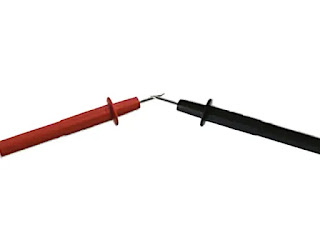How do you check continuity with a multimeter?

Now we try to understand what is the reason behind this beep sound. Why do we need to do this continuity testing? To understand the beep testing reason we have a house wiring continuity check example here in this blog post. when we are checking any wire with a multimeter and you want to check whether the wire is broken in-between. whether the wire is broken from the inside. you will not be able to find it out looking at it from the outside. most peoples ask

Before you know how to use an analog multimeter continuity mode let me tell you what is continuity mode on a digital multimeter and how to use beep testing to check mobile phone PCB

This video on the Sanwa digital multimeter explains to you how to check the Ground Line on a mobile PCB

#### How do I test my house wires with a multimeter?

How to check continuity in a long wire. To check a wire you need to check the current continuity on it. that's why we use beep (buzzer) or continuity mode on the analog meter. to check the wire we set the meter knob to buzzer mode.House wiring continuity check

Then we touch meter both probes on both ends of the wire. which means you touch black to one end of the wire and red to another end of the wire.How to check for a broken wire with a multimeter

If you hear a beep sound which means that the circuit is not open the wire is proper. typically broken wires are called open wires in the mobile repairing field.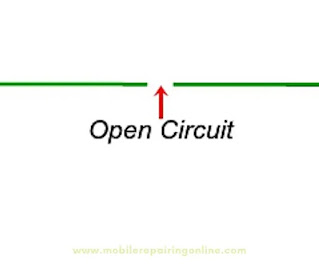Uses of multimeter

But, if you don't hear a beep it means the wire is broken from the inside. the method for the analog multimeter continuity test for cables you see in this continuity test results is the same. you can use it for any wire testing. the method is the same while doing tracing on a printed circuit board (PCB) to check whether two points are connected properly. when setting the analog meter knob on beep mode. testing anything it doesn't matter for you to place the meter negative probe to the negative and positive probe to the positive lead.

## Cell Phone Repair School

A new mobile cell phone repair business startup wants to learn how to read multimeters that's why cell phone repair schools help them to know the working principle of multimeters. now in Canada learning how to use an analog multimeter has been a popular search term for learning the multimeter working principle to repair mobile cell phones. cell phone technician training school near you can educate you on the digital multimeter working principle and help you read and understand diagram analog multimeter drawing. Because the teacher in the cell phone repair school understands the block diagram of analog multimeter which is why I advise cellphone repair business entrepreneurs to enroll in mobile phone repair training classes to gain in-depth information on the following topics.
• what is analogue multimeter
• analog meter definition
• analog multimeter circuit diagram
• how to use a analog multimeter tester
• how to read analogue multimeter
• how to read analog multimeter ohms
I came across quite several questions about Analog Multimeter so that's why I am publishing this blog post. I hope you like it.

Do you want to know how to use continuity mode on a digital multimeter to check the Ground Line on a Phone PCB read this blog post

1.Dear Sir,
Your education on mobile phones is Powerful and all electronic technicians are befitting

2.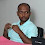3.